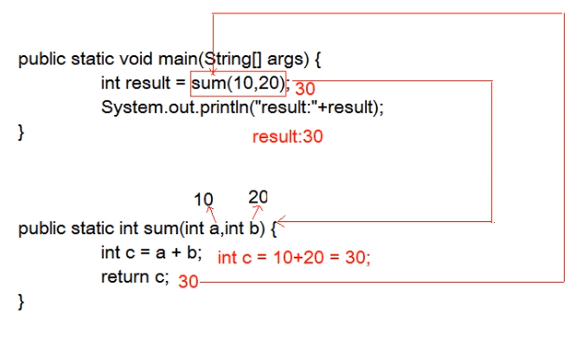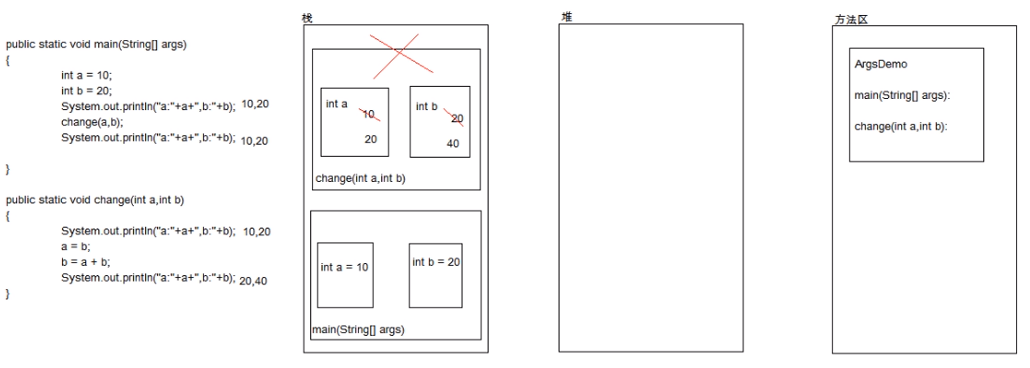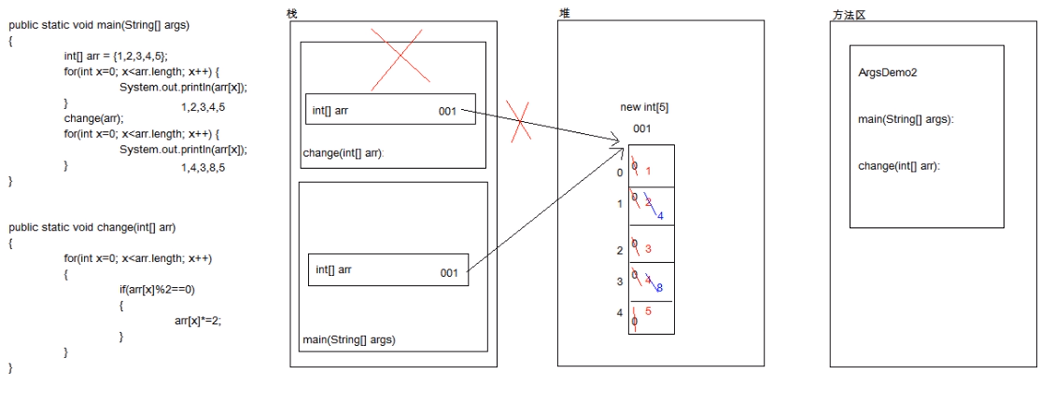# JAVA part 5：方法1218-马同学

,

# JAVA part 5：方法

## 方法

    修饰符 返回值类型 方法名(参数类型 参数名1, 参数类型 参数名......){
方法体;
return 返回值;
}


tips:
1.修饰符：使用 public static即可
2.返回值类型：用于限定返回值的数据类型
3.方法名：方便调用方法
4.参数类型：用于限定调用方法时传入的数据的数据类型
5.参数名：用于接收调用方法时传入的数据的变量
6.方法体：完成特定功能的代码
7.return 返回值：结束方法并把返回值传给调用者

### 方法调用

1.单独调用（没有意义）
2.赋值调用（推荐）
3.输出调用（不推荐）

package cases;
public class x21 {
public static void main(String[] args) {

//调用方法不仅仅要看方法名，还要看方法的参数（个数和类型）

//单独调用
sum(10, 20);

//赋值调用
int result = sum(10, 20);
System.out.println("result:"+result);

//输出调用
System.out.println("result:"+result);
}
public static int sum(int a, int b){
int c = a+b;
return c;
}
}### 方法重载

1.方法名相同；
2.参数列表不同：
①参数的个数不同
②参数的数据类型不同

package Day_5;
public class test2 {
public static void main(String[] args) {

int x = sum(1,2);
System.out.println(x);
System.out.println("---------------");

int y = sum(1,2,3);
System.out.println(y);
System.out.println("---------------");

double z = sum(1.5,2.5);
System.out.println(z);
System.out.println("---------------");
}
// 定义了一个有两个参数的sum方法
public static int sum(int a, int b){
int c = a + b;
return c;
}
// 定义了一个有三个参数的sum方法
public static int sum(int a, int b, int c){
int d = a + b + c;
return d;
}
// 定义了一个有两个参数的sum方法
public static double sum(double a, double b){
double c = a + b;
return c;
}
}


### 方法参数是基本类型：

package Day_5;
public class test4 {
public static void main(String[] args) {

int a = 10;
int b = 20;
System.out.println("a:"+a+"b:"+b);
change(a,b);
System.out.println("a:"+a+"b:"+b);
}

public static void change(int a, int b){
System.out.println("a:"+a+"b:"+b);
a = b;
b = a+b;
System.out.println("a:"+a+"b:"+b);
}
}tips:

①形式参数：用于接收实际参数的变量
②实际参数：实际参与运算的数据

### 方法参数是应用类型：

package Day_5;
public class test3 {
public static void main(String[] args) {

int[] arr = {1, 2, 3, 4, 5};
for(int x=0; x< arr.length; x++){
System.out.println(arr[x]);
}

change(arr);
for(int x=0; x<arr.length; x++){
System.out.println(arr[x]);
}
}

public static void change(int[] arr){
for(int x=0; x<arr.length; x++){
// 如果数组中的元素是偶数，值就变成了以前的2倍
if(arr[x]%2 == 0){
arr[x] *= 2;
}
}
}
}tips：
1.基本数据类型作为参数传递，形式参数的改变不影响实际参数
2.应用数据类型作为参数传递，形式参数的改变直接影响实际参数

### 评论 抢沙发### 觉得文章有用就打赏一下文章作者

#### 支付宝扫一扫打赏#### 微信扫一扫打赏Vieu3.3主题Q Q 登 录